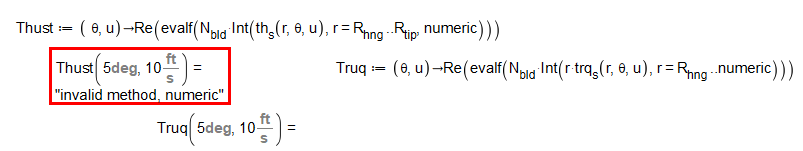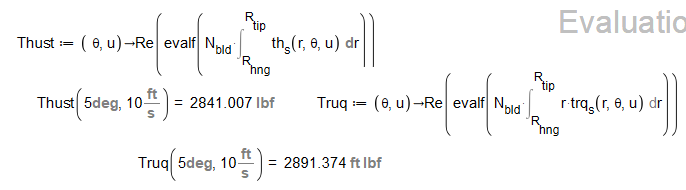## 35 Reputation

1 years, 236 days

## Flow file attached...

Attached is my Maple Flow file Final_helicopter_model_C.pdf

Als an aside, I'm trying to learn Flow as an alternative to Mathcad.  (I used Mathcad for many years, starting when Maple was the symbolic engine.)  Mathcad has recently shifted to an annual fee; this (along with the shift to Mathcad Prime which is a poor substitute that still, after years of development, lacks many of the features of the original, is driving long-time Mathcad users to search for a replacement.  There is still a free version of Mathcad Prime (stripped of "prermium" features) that is surprisingly capable.  The Mathcad Prime Express (aka "free') version of the completed file is MCAD_helicopter_final.pdf.

## Thanks! That was was a lot of work...

Let me take this into Flow and see what I can do.

## NACA 0012 drag vs angle...

file attached.  extension changed from .csv to .xlsx to upload NACA0012_CD_alpha.xlsx

## But, but, but . ....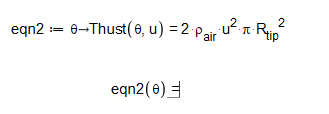Picture of Maple Flow after 5 minutes of "Evaluating".  (I don't think it's going to ever finish!)

Also, after 5 minutes:And, for those who suggest: "Or complete the attached Maple sheet, MP_234625_1.mw"

Sorry, I don't have Maple!  I'm using Maple Flow.  Files with extension .mw open, but as some strange text file.

## Copy paste...

tm := Vector(10, 0, orientation = row)          set up time vector for execution times

N__bld := 4;                                                   four blades on the helicopter

D__rtr := 30*ft;    `R__tip`:=`D__rtr`/(2)=;     chd:=8in;     R__hng := ft;

rho__air:=ThermophysicalData:-Atmosphere(10,density,useunits)=;
`a__air`:=ThermophysicalData:-Atmosphere(10, speedofsound, useunits)=;

M__tip := 0.62

omega:=(`M__tip`*`a__air`)/(`R__tip`)=;

`Cl__&alpha;` := 0.1/deg;     C__L := alpha -> alpha*`Cl__&alpha;`        coefficient of lift

data := Import("C:/Users/fkohl/Desktop/excess mcad/NACA0012 CD alpha.csv");
Data := convert(data, 'Matrix');
alp := Column(Data, 1)*deg;  cd := Column(Data, 2);
ft := Statistics:-PolynomialFit(6, Data, x);
C__D := alpha -> eval(ft, x = alpha);

alpha__i := (r, theta, u) -> theta - arctan(u/(omega*r)) - 8*deg*r/R__tip + 6*deg;

lf__s := (r, theta, u) -> 1/2*rho__air*chd*(omega^2*r^2 + u^2)*C__L(alpha__i(r, theta, u));
dr__s := (r, theta, u) -> 1/2*rho__air*chd*(omega^2*r^2 + u^2)*C__D(alpha__i(r, theta, u));
th__s := (r, theta, u) -> evalf(lf__s(r, theta, u)*cos(alpha__i(r, theta, u)) - dr__s(r, theta, u)*sin(alpha__i(r, theta, u)));
trq__s := (r, theta, u) -> evalf(dr__s(r, theta, u)*cos(alpha__i(r, theta, u)) + lf__s(r, theta, u)*sin(alpha__i(r, theta, u)));
Thust := (theta, u) -> N__bld*int(th__s(r, theta, u), r = R__hng .. R__tip, numeric);
UD := u -> 2*rho__air*u^2*Pi*R__tip^2;

eqn2 := theta -> Thust(theta, u) = 2*rho__air*u^2*Pi*R__tip^2;
U := theta -> fsolve(eqn2(theta), u = 0*ft/s .. 100*ft/s);

Above was generated by triple-clicking the Flow equation,  copying and pasting in this document.

As you can see, The equation for thrust (Thust) is built on a whole pile of other functions.  That's why it's not easily explained.

## Without Units??...

Without Units?  On something this complicated unit balance is essential.  If I had to do it manually, I could use EXCEL! ;-(

I will assume that you don't have Flow, only Maple, since Flow is relatively new.

I chose a NACA 0012 airfoil, it's a basic standard with well documented properties.  coefficient of lift is essentially linear in angle, I downloaded a drag coefficient data set and fit a sixth order polynomial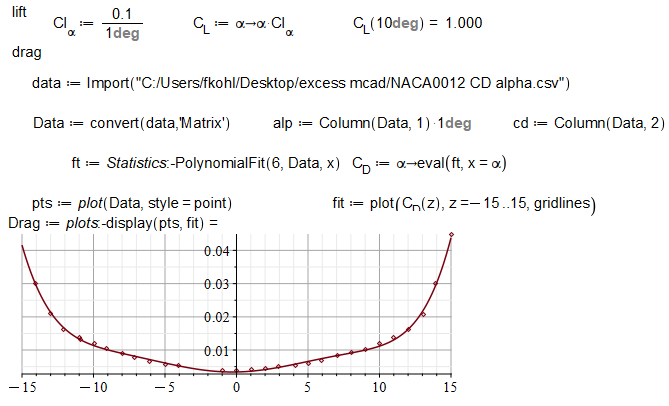Lift and drag of an airfoil section is then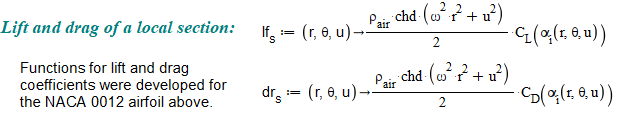where local angle of attack depends on set angle (theta), downflow velocity (u) and the radial position along the twisted blade: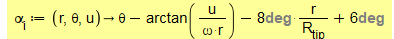But lift and drag align with the "wind vector," so we must do a coordinate rotation into shaft axis: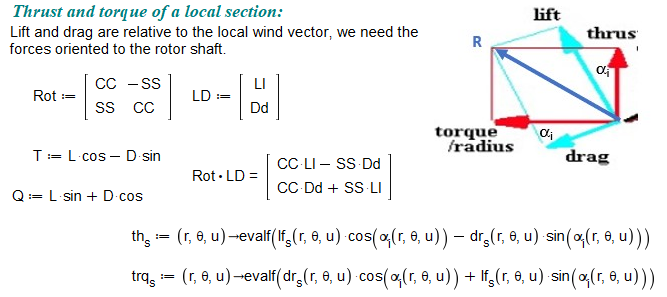That's for a local section,  Thrust (as function "Thust") is the integral alond the blade: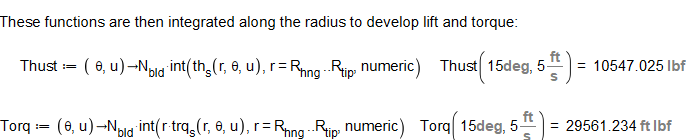So that's how you compute thrust (Thust), which we now must solve for the momentum balance: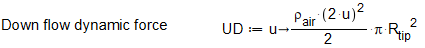Which I first tried to find downflow using fsolve: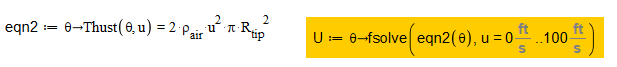And that's how I got here!

## Why—Maple Flow...

I'm working in Maple Flow, not Maple.  I could upload that sheet, but its already essentially here in another post.

## Iteration example...

Thank you for the response!  Some explanation is in order.  This is intended to be a math model of a helicopter rotor.  The thrust (Thust) of the helicopter is developed by taking the lift and drag coefficients of the airfoil chosen and the blade angle, rotating the resulting forces from airflow aligned coordinates to rotor shaft axis and integrating along the blade radial station to get the thrust component ofeach blade.  Times the number of blades is total thrust.

The problem is this:  When the rotor generates thrust it creates a downwash, anyone watching a helicopter can see the air flow under the rotor.  That downwash changes the effective angle of attack of each blade section.  Which, of course, changes the lift and drag produced.  So downwash flow ("u" in my notation) depends on thrust, which is calculated by knowing both "u" and "theta", the set geometric angle of the blade.  We can do a momentum balance  and equate the rotor thrust to the flow thru the rotor; this is what the function "Fn" is--the difference between Thust(theta, u) and the total flux through the rotor.  I can plot Thust as a function of u for a given blade angle theta, and plot the flux through the rotor (the two terms of Fn):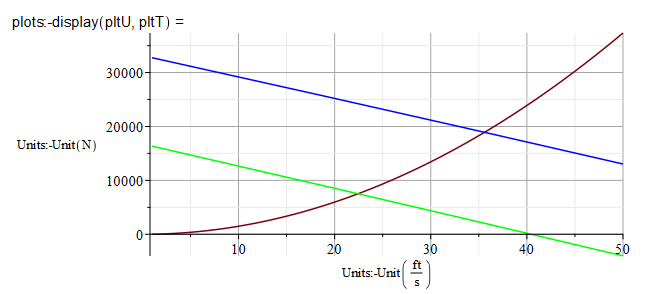The (nearly) straight lines are Thust plotted against u for theta = 5 deg (green) and 10 deg (blue) while the momentum flux is the red curve.  The intersection of these two curves is the true downflow velocity "u" for a given blade angle setting.  So If I find the roots of Fn, I have my downflow.  I can then solve for thrust (and torque) set by blade angle only.

Attempts to fsolve Fn, even to plot Fn, in Maple Flow end up with the program spinning its' wheels until my patience or the computer's runs out.  But Fn can be evaluated at a single point: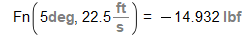, and DFn (it's finite element derivative) can too: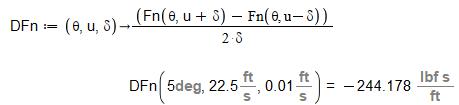So a "home built" root finder seemed possible, and it is: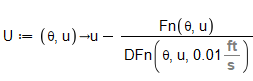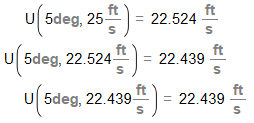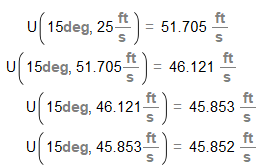But I need help automating this.

## Why not fsolve?...

I'm building a finite difference equivalent to derivative because I'm not sure that taking Maple Flow's derivative isn't part of the problem.  And the value of delta (0.01) is a "first start," to be modified later if necessary.

Please see the expanded comments to Rouben Rostamian 6539 below for further explanation.

## Significant changes...

I got a notification that 2022.1 was available last week; installation of the update was  smooth and prompt!  Image paste is a significant improvement, thanks!!  I'll be investigating the other changes as I progress.

## Sorry!...

I'm sorry, thank you for your help, I should have replied to your earlier input.  I'm new to this forum--that's no excuse for my lack of response!

I've been flogging this problem for some time now, gaining ground by small steps as I learn this new (to me) software. To answer your question by your numbers:

1. Singularities:  No, these functions are all smooth.  These are lift and drag of an airfoil section--the only time they might approach singularity would be if the airfoil stalled and that has been carefully avoided.
2. Loss of precision:  I tried the epsilon option, did not see an effect.
3. Massively complicated:  Well, maybe.  The calculation begins with the lift and drag at a particular radial station.  These are rotated from local airfoil coordinates to rotor shaft coordinates.  These are then integrated along the blade radial stations to develop rotor thrust and torque.  So there is a complicated sequence of calculations involved.  But the inclusion of int(numereic) in the calculation has helped.
4. Inadvertant symbolic calculations:  This has possibilities.  So how do I find those and shut them down?

I put up a post yesterday with two files attached.  Shortly I got a message from acer 26226 that it had been deleted with an admonition not to spawn a second thread.  (Again, my apologies--I'm new here.)  Unfortunately those files showed what progress I had made.

The first, for_MaplePrime.zip, contains a new version of my Maple Flow file, and a pdf of the Mathcad file of the same calculation.  I've managed (I think) to implement time stamping thru the files to illustrate the problem.  The Flow file isn't as complete as the other  because it never evaluates its' last calculation, so I have not continued the development.  The pdf of the Mathcad file shows the entire operation.

The second file, Final_model_helicopter_MCAD.zip, (for the adventerous) is the actual Mathcad file.  If you have a version of Mathcad Prime (they'll give you a free copy) that's version 4.0 or newer you may see for yourself.  Download the file then change the extension from "zip" to "mcdx".

## Hovering helicopter...

Attached (I hope) is a zip file with a pdf printout of a Mathcad file that I've been trying to replicate in Flow.for_MaplePrime.zip

## Making it stop!...

In Flow 2022.0, I've discovered that a worksheet that is "Evaluating" can be interrupted.  If I close the offending sheetby clicking on the x, the process will ask if I want to save (and it WILL save any changes) and terminate.  I'm then free to reopen that document and try again.

Not great, but better than trying to use the task manager.  (Note that I've had that route fail--the sheet refuses to be closed and a "power off reset" was required.)

## Well, maybe, but not yet...